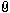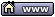flat assembler
Message board for the users of flat assembler.flat assembler > Projects and Ideas > [DEAD] X to the power of...well Y
Author
 ThreadJoined: 25 Sep 2003
Posts: 2145
Location: Estonia
Hi,
another program that demonstrates my bad programming habits - it was the time when I made a lot of unuseful comments like
Code:
;this line increases eax by one

but if you don't let it disturb, you might even find something useful out of it.

What it does it take x^y like 3^4=3*3*3*3=81 etc. but it can handle large numbers like 2^131072 and still show accurate results - I might reprogram it some day to output to a file or a text-edit boxDescription: Power is in YOUR hands :) Just exponentiates two numbers - change the code to suit your exponent sign requirements like "y" or "x", maybe "E" :DDownload Filename: Power.7z Filesize: 6.67 KB Downloaded: 483 Time(s)

_________________
My updated idolhttp://www.agner.org/optimize/

Last edited by Madis731 on 08 Sep 2006, 10:00; edited 1 time in total28 Feb 2006, 20:56
 Madis731 Joined: 25 Sep 2003 Posts: 2145 Location: Estonia Btw, I revised the idea of using logaritms and they tend to be useful. The way logarithms works is really easy: 5*6=30 => log5+log6=log30 a*b=c? => c=10^(loga+logb) 5^6=15625 => log5*6=log15625 a^b=c? => c=10^(loga*b) In floating point world 80-bit is suggested and if you are afraid of losing precision you can always half the numbers. 2^128=2^(16+16+16+16+16+16+16+16)=2^16*2^16*2^16*2^16*2^16*2^16*2^16*2^16 Then the propagating error will reset every time when you round the result of the 2^16 before you multiply it with another 2^16.26 Apr 2006, 11:35
Mota

Joined: 29 Dec 2004
Posts: 22
Well, I've just devised a simple recursive pow function that works exclusively for integers. It is guaranteed to work faster than your average loop, because it splits an equation in the most efficient way: down the middle.

Code:
pow: ; I: eax = x, ecx = y / O: eax = x^y

or ecx, ecx
jnz .cont0
mov eax, 1
ret               ; x^0 = 1

.cont0:
cmp ecx, 1
jnz .cont1
ret               ; x^1 = x

.cont1:
cmp ecx, 2
jnz .cont2
mul eax, eax      ; x^2 = x*x
ret

.cont2: ; Okay, what happens is that (x^y = x^(y >> 1)^2 * x^(y & 1)),
;  therefore minimizing the number of necessary operations.

test ecx, 1
jz .no_one

push ecx eax
shr ecx, 1
call pow
mul eax, eax
mul eax, [esi]
pop ecx ecx
ret

jmp .cont
.no_one:
push ecx
shr ecx, 1
call pow  ; (x^(y/2) * x^(y/2)) = (x^(y/2))^2
mul eax, eax  ; so there is no need to recalculate the value.
pop ecx
ret

This was good exercise. Another one for the code bank.08 May 2006, 23:14Madis731 Joined: 25 Sep 2003 Posts: 2145 Location: Estonia You mean: Code:pow: ; I: eax = x, ecx = y / O: eax = x^y or ecx, ecx jnz .cont0 mov eax, 1 ret ; x^0 = 1 .cont0: cmp ecx, 1 jnz .cont1 ret ; x^1 = x .cont1: cmp ecx, 2 jnz .cont2 mul eax ; x^2 = x*x ret .cont2: ; Okay, what happens is that (x^y = x^(y >> 1)^2 * x^(y & 1)), ; therefore minimizing the number of necessary operations. test ecx, 1 jz .no_one push ecx eax shr ecx, 1 call pow mul eax mul dword[esp] pop ecx ecx ret ; jmp .cont .no_one: push ecx shr ecx, 1 call pow ; (x^(y/2) * x^(y/2)) = (x^(y/2))^2 mul eax ; so there is no need to recalculate the value. pop ecx ret10 May 2006, 08:41
Mota

Joined: 29 Dec 2004
Posts: 22
No, I mean exactly what I wrote. What you wrote won't even compile.

Anyway, peace out.11 May 2006, 22:12donkey7

Joined: 31 Jan 2005
Posts: 127
Location: Poland, Malopolska
slightly shorter version:
Code:
format pe gui
power   equ 7
base    equ 5

mov     ecx,power
mov     eax,base
mov     ebx,1
.loop:
shr     ecx,1
jnc     .skip
imul    ebx,eax
.skip:
imul    eax,eax
or      ecx,ecx
jnz     .loop
int3

result in ebx. should work :)
btw: algorithm taken from clr's 'introduction to algorithms'. interesting book!

_________________
Keep coding!16 May 2006, 17:19vid Verbosity in development Joined: 05 Sep 2003 Posts: 7108 Location: Slovakia hmmm... is this really a project?08 Sep 2006, 08:26
 Madis731 Joined: 25 Sep 2003 Posts: 2145 Location: Estonia Well, noa demonstration of some ideas at most...08 Sep 2006, 09:59
 Display posts from previous: All Posts1 Day7 Days2 Weeks1 Month3 Months6 Months1 Year Oldest FirstNewest FirstJump to: Select a forum Official----------------AssemblyPeripheria General----------------MainDOSWindowsLinuxUnixMenuetOS Specific----------------MacroinstructionsCompiler InternalsIDE DevelopmentOS ConstructionNon-x86 architecturesHigh Level LanguagesProgramming Language DesignProjects and IdeasExamples and Tutorials Other----------------FeedbackHeapTest Area

Forum Rules:
 You cannot post new topics in this forumYou cannot reply to topics in this forumYou cannot edit your posts in this forumYou cannot delete your posts in this forumYou cannot vote in polls in this forumYou cannot attach files in this forumYou can download files in this forum